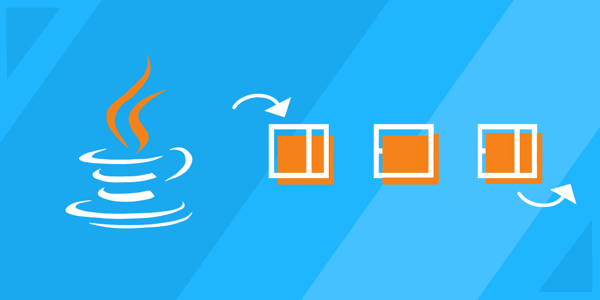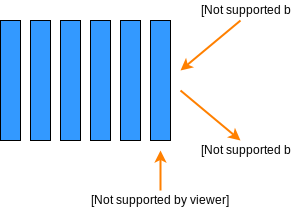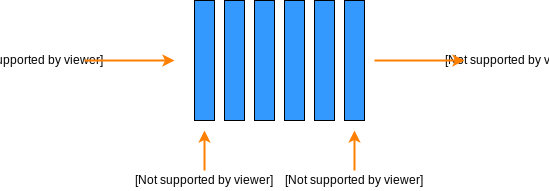Home/Blog/Tutorials & Guides/Data structures 101: How to use stacks and queues in Java

# Data structures 101: How to use stacks and queues in Java

Sep 15, 2020 - 9 min readJerry EjonaviMastering data structures is non-negotiable. Efficient data structures help execute effective programs. Today, many programming roles require great knowledge of data structures. They are also a fundamental part of coding interviews.

Stacks and queues are linear data structures that follow a particular order to add or remove entities. In this article, you will be introduced to stacks and queues. We will highlight their uses, functionalities, and show you how to implement these data structures in Java.

Today, we will cover:

## What is a Stack?

In programming, a stack is an abstract, linear data type with a predefined capacity (or boundary). It follows a particular order for adding or removing elements. Linear data structures organize their components in a straight line, so if we add or remove an element, they will grow or shrink respectively.Insertion and deletion happen on the same end

Let us conceptualize stacks using a stack of plates placed in a box. The first plate placed in the stack (the plate at the bottom of the stack) will be the last one to be removed, and the plate added last would be the first to be removed.

This is called the Last In First Out (LIFO) or First In Last Out (FILO) ordering.

Stacks are used in a variety of ways when we code. We use stacks to implement functions, parsers, expression evaluation, and some algorithms. Stacks are great for processing nested structures, so they are important for understanding recursion.

A simple real-world application of a stack is reversing a string letter by letter. Another good example of a data stack is the undo and redo function on a computer or text editor. Undo removes your most recent change, and redo builds upon already existing changes.

### How do Stacks work?

The implementation of stacks is relatively easy. The functionality depends on the pop and push method, as you can see from the illustration above. The pop method removes or deletes elements from the stack, while the push method adds items to the stack.

When an element is inserted into a stack, it takes the top position and the variable storing this position points to the number below it. The top variable should be updated anytime an element is inserted or removed from it.

Note: What’s important to remember is that insertion and deletion happen on the same end of a Stack.

Later in this article, we will learn how to manually implementing the Stack data structure in Java.

## What is a Queue?

A queue is a lot like a stack. A Queue is also a linear structure that follows a First In First Out (FIFO) order, but they differ in how elements are removed. Queues are open from both ends: one end for inserting data (enqueue), and the other end for removing data (dequeue). A stack is only open from one end.

Simplied: for a stack we remove the most recently added element, but for a queue, we remove the “oldest” element.Insertion and deletion happen on different ends

When it comes to queues, think of a check out counter at your favorite grocery store. The first person in the checkout line will be attended to first before others, and the last person in line will be attend to last. This is how a queue works. It has two ends, front and rear. Elements enter from the rear and leave from the front.

### Types of Queues

There are three common types of queues you can expect to encounter. So far, we learned about the Linear Queue. The other two queues are:

1. Circular Queue: In a circular queue, the last element is connected to the first element to form a circle. Initially, the front and rear parts of the queue point to the same location, but they move apart as more elements are inserted into the queue. A real-life example of a circular queue is an automated traffic signal system.

2. Priority Queue: In priority queues, elements are sorted based on priority. The most important element appears first, and the least important appears last. Priority queues are used in operating systems for load balancing to determine which programs should be given more priority.

## Pros and Cons of Stack and Queues

### Stacks

Pros

• It is easy to implement and logical for beginners
• It allows you to control how memory is allocated
• Easier to use than queues

Cons

• Neither flexible nor scalable

### Queues

Pros

• Queues are flexible.
• They can handle multiple data types.
• Data queues are fast and optimized

Cons

• Inserting/ removing elements from the middle is complex.
• Queues are not readily searchable

## Essential Operations for Stacks & Queues

A typical stack must contain the following methods:

• pop(): this method removes an element from the top of the stack and returns it.
• push(): this method adds an element to the top of the stack.

A queue allows for the following operations:

• enqueue(): this method adds an element to the end/rear of the queue
• dequeue(): this method removes an element from the front of the queue
• top(): returns the first element in the queue
• initialize(): creates an empty queue

From there we can apply all sorts of methods for more functionality and information retrieval:

• top(): returns the element most recently added to the stack
• intStack.peek(): returns the top of the stack without removing an element
• poll(): removes the head of a queue and returns it
• size(): returns the size of the queue as number of elements
• isEmpty(): returns true if the stack/queue is full
• isFull(): returns true if the stack/queue is full

### Master data structures for coding interviews.

Learn data structures and algorithms in Java without scrubbing through videos. Educative’s text-based courses are easy to skim and feature live coding environments, making learning quick and efficient.

Data Structures for Coding Interviews in Java

## How to implement a Stack in Java

Every programming language comes with basic functionality for stacks. However, in Java, the stack data type is an Adapter class. This means that it is built on top of other data structures. Therefore, it can be implemented using an Array, Vector, Linked List, or any other collection.

Note: Stacks are generally implemented using Arrays because it takes less memory.

Irrespective of the underlying data structure or programming language used, stacks must implement the same basic functionalities. In Java, you can import a pre-built class of stack or manually implement a stack and extend its functionality. To implement the built-in Stack class, we use the java.util package using the following import statement:

import java.util.*;
// or
import java.util.Stack;


Once we import the package, we can create a stack object like this:

Stack mystack = new Stack();


Then, we add elements to the stage. We could, for example, add integers using push().

Stack<Integer> myStack = new Stack<>();
myStack.push(5);
myStack.push(6);
myStack.push(7);


The basic syntax will look like this:

public class Stack <V> {
private int maxSize;
private int top;
private V arr[];


To make a stack manually, we construct a class of Stack and create an instance. This class has the following three data members:

• An array that will hold all the elements
• The size/boundary of this array
• A variable for the top element of the stack

The following code shows how to construct the Stack class:

main.java
Stack.java
class StackDemo {    public static void main( String args[] ) {        Stack<Integer> stack = new Stack<Integer>(10);        System.out.print("You have successfully created a Stack!");    }}

Before adding the push and pop methods into this code, we need to implement some helper methods. Helper methods keep the code simple and understandable. Here’s the list of helper functions that we will implement in the code below:

• isEmpty()
• isFull()
• top()

Here is the code for stacks with the new helper methods.

main.java
Stack.java
public class Stack <V> {    private int maxSize;    private int top;    private V array[];    /*    Java does not allow generic type arrays. So we have used an    array of Object type and type-casted it to the generic type V.    This type-casting is unsafe and produces a warning.    Comment out the line below and execute again to see the warning.    */    @SuppressWarnings("unchecked")    public Stack(int max_size) {        this.maxSize = max_size;        this.top = -1; //initially when stack is empty        array = (V[]) new Object[max_size];//type casting Object[] to V[]    }    //returns the maximum size capacity    public int getMaxSize() {        return maxSize;    }    //returns true if Stack is empty    public boolean isEmpty(){        return top == -1;    }    //returns true if Stack is full    public boolean isFull(){        return top == maxSize -1;    }    //returns the value at top of Stack    public V top(){        if(isEmpty())            return null;        return array[top];    }}

If your output returns true for isEmpty() and false for isFull(), it means that these helper functions are working properly! Now, take a look at this extended code with push and pop functions added to the definition of Stack class. We will try to add and remove some elements from this stack.

main.java
Stack.java
public class Stack <V> {    private int maxSize;    private int top;    private V array[];    /*    Java does not allow generic type arrays. So we have used an    array of Object type and type-casted it to the generic type V.    This type-casting is unsafe and produces a warning.    Comment out the line below and execute again to see the warning.    */    @SuppressWarnings("unchecked")    public Stack(int max_size) {        this.maxSize = max_size;        this.top = -1; //initially when stack is empty        array = (V[]) new Object[max_size];//type casting Object[] to V[]    }    //returns the maximum size capacity    public int getMaxSize() {        return maxSize;    }    //returns true if Stack is empty    public boolean isEmpty(){        return top == -1;    }    //returns true if Stack is full    public boolean isFull(){        return top == maxSize -1;    }    //returns the value at top of Stack    public V top(){        if(isEmpty())            return null;        return array[top];    }    //inserts a value to the top of Stack    public void push(V value){        if(isFull()) {            System.err.println("Stack is Full!");            return;        }        array[++top] = value; //increments the top and adds value to updated top    }    //removes a value from top of Stack and returns    public V pop(){        if(isEmpty())            return null;        return array[top--]; //returns value and top and decrements the top    }}

In the code output, you can see that the elements popped out of the stack in the exact reverse order as they were pushed in. That means our stack works perfectly.

## How to Implement a Queue in Java

The most common queue implementation is using Arrays, but it can also be implemented using Linked Lists or by starting from a Stack. We can import the queue interface with this command:

import java.util.queue;
// or
import java.util.*;


We then create a queue interface with the following statement, which creates a linked list for our queue, and provide values:

Queue<String> str_queue = new LinkedList<> ();


Let’s see a manual example of a Queue class with an integer data type and create an instance. The class would hold 5 data members to hold the following information:

• An array that will contain all the elements
• The maxSize is the size of this array
• The front element of the Queue
• The back element of the Queue
• The currentSize of elements in the Queue

The code given below shows how to construct the Queue class:

main.java
Queue.java
class QueueDemo { 	public static void main(String[] args) {		Queue<Integer> queue = new Queue<Integer>(5);        System.out.print("You have successfully created a Queue!");	}}

Before adding the enqueue and dequeue methods into this class, we need to implement some helper methods. The aforementioned helper method would be used here. Now run the following code and see if the helper function outputs correctly.

main.java
Queue.java
class QueueDemo {	public static void main(String[] args) {		Queue<Integer> queue = new Queue<Integer>(5); //create the queue            if(queue.isEmpty())        System.out.print("Queue is empty.");        else        System.out.print("Queue is not empty.");                System.out.printf("%n");                if(queue.isFull())        System.out.print("Queue is full.");        else        System.out.print("Queue is not full.");	}}

For the above code, since the queue is empty, isEmpty() should return true, and isFull() should return false. Now, we will extend this code with the enqueue method to add elements and the dequeue method to remove elements from the queue.

main.java
Queue.java
class QueueDemo {	public static void main(String[] args) {		Queue<Integer> queue = new Queue<Integer>(5);		//equeue 2 4 6 8 10 at the end        queue.enqueue(2);		queue.enqueue(4);		queue.enqueue(6);		queue.enqueue(8);		queue.enqueue(10);        //dequeue 2 elements from the start		queue.dequeue();		queue.dequeue();		//enqueue 12 and 14 at the end        queue.enqueue(12);        queue.enqueue(14);        System.out.println("Queue:");        while(!queue.isEmpty()){                System.out.print(queue.dequeue()+" ");            }	}}

Take a look at the output of the code and take note that the elements are enqueued in the back and dequeued from the front. This means that our queue works perfectly.

## What to learn next & interview questions

You made it to the end of this article. Congratulations! I hope you now have a good foundation of how stacks and queues data structures work. There’s so much more to learn to master queues and stacks in Java. Here are some of the common interview challenges for these data structures:

• Implement a Queue using Stacks
• Generate Binary Numbers from 1 to n using a Queue
• Evaluate Postfix Expressions using Stacks
• Implement two stacks using one array
• Reversing the First k Elements of a Queue
• Create Stack where min() gives minimum in $O(1)$
• and more

To get started on these questions, check out Educative’s course on Data Structures for Coding Interviews in Java. It will give you a detailed explanation of all common Java data structures with solutions to real-world data structure problems. By the end, you’ll be an intermediate Java programmer, ready to conquer coding interviews!

Happy learning!